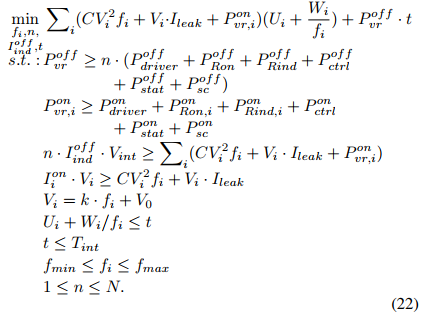# Problem with CVX

Hi to all,
i am new in convex optimization and CVX, so excuse me for simple and silly question. i want to solve below convex optimization problem with CVX.in above problem, there is an in inequality:
I(on) * Vi >= C * (Vi ^ 2) * Fi + Vi . Ileak
in the above inequality there is I(on) * Vi expression that both of Vi and I(on) should be variable and as you now CVX dose not permit me to done this and it won’t accept product of two variable. now, there is any way to solve this problem??

I can’t read that problem clearly enough to confidently know what the optimization variables are (i.e., the variables listed below “min” ) vs. input data. However, I don’t see I_i^{on} listed among the optimization variables, although I_{ind}^{off} is.

In any event, how do you know this is a convex optimization problem? Note that this is crucially dependent on what the optimization variables are vs. what the input data is, and possibly on the values of the input data.

If I^{on} and I^{off} are binary variables meant to convey on or off status, then they should not be multiplied against a continuous variable, even though that is mathematically correct. Rather, the product should be “linearized” as shown in section 2,8 of https://www.fico.com/en/resource-download-file/3217 ., which will make it MIDCP compliant, and enterable in CVX.